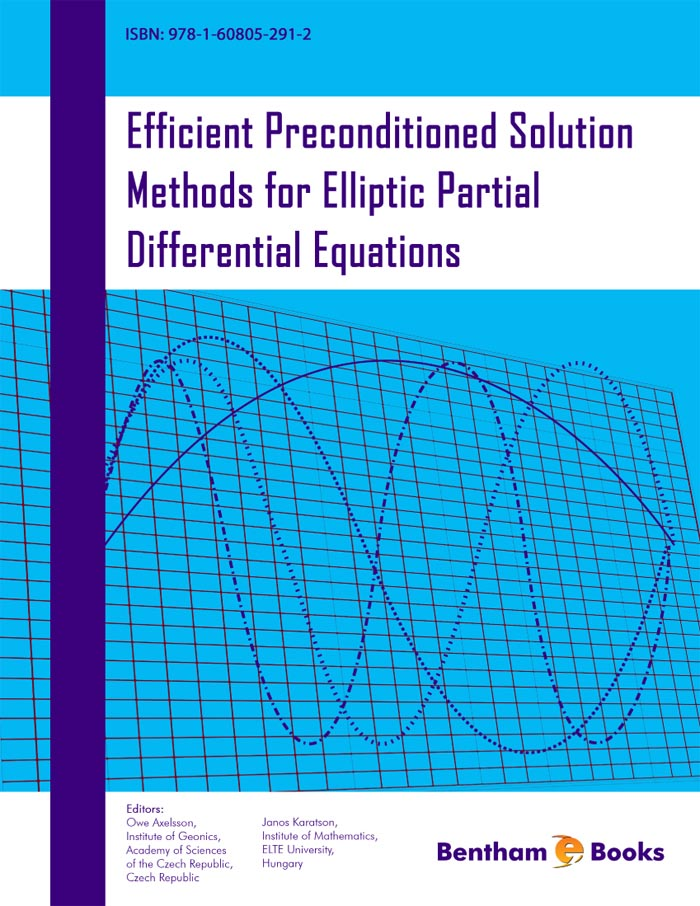### Robust Multilevel Preconditioning Methods

#### Abstract

&lt;p&gt;Preconditioners based on various multilevel extensions of two-level finite element methods (FEM) lead to iterative methods which have an optimal order computational complexity with respect to the size of the system. The methods can be based on block matrix factorized form, recursively extended via certain matrix polynomial approximations of the arising Schur complement matrices, or on additive, i.e., block diagonal form, using stabilizations of the condition number at certain levels. In this survey, the method in focus is the Algebraic MultiLevel Iteration (AMLI). It was introduced and studied by Axelsson and Vassilevski for the case of isotropic elliptic problems in [1,2], and is referred to as AMLI. The further development of robust AMLI methods for elliptic problems is systematically discussed in the recently published monograph .&lt;/p&gt; &lt;p&gt;While a huge amount of papers are dealing with the solution of FEM elliptic systems, the related time dependent problems are much less studied. A comparative analysis of robust AMLI methods for elliptic and parabolic problems is presented in this chapter. A unified framework for both Courant conforming and Crouzeix-Raviart nonconforming linear finite element discretizations in space is used. The considered multiplicative AMLI methods are based on an approximated block two-by-two factorization of the original system matrix. A key ingredient for the efficiency of the AMLI preconditioners is the quality of the utilized block two-by-two splitting, quantified by the so-called Cauchy- Bunyakowski-Schwarz (CBS) constant, which measures the abstract angle between the two subspaces, associated with the two-by-two block splitting of the matrix. All presented methods lead to preconditioners with spectral equivalence bounds which hold uniformly with respect to both the problem and discretization parameters, and optimal total computational complexity of the related Preconditioned Conjugate Gradient (PCG) or Generalized Conjugate Gradient (GCG) solvers. The theoretical results are supported by numerical tests with an emphasis on the case of nonconforming FEM systems.&lt;/p&gt;

Total Pages: 3-22 (20)